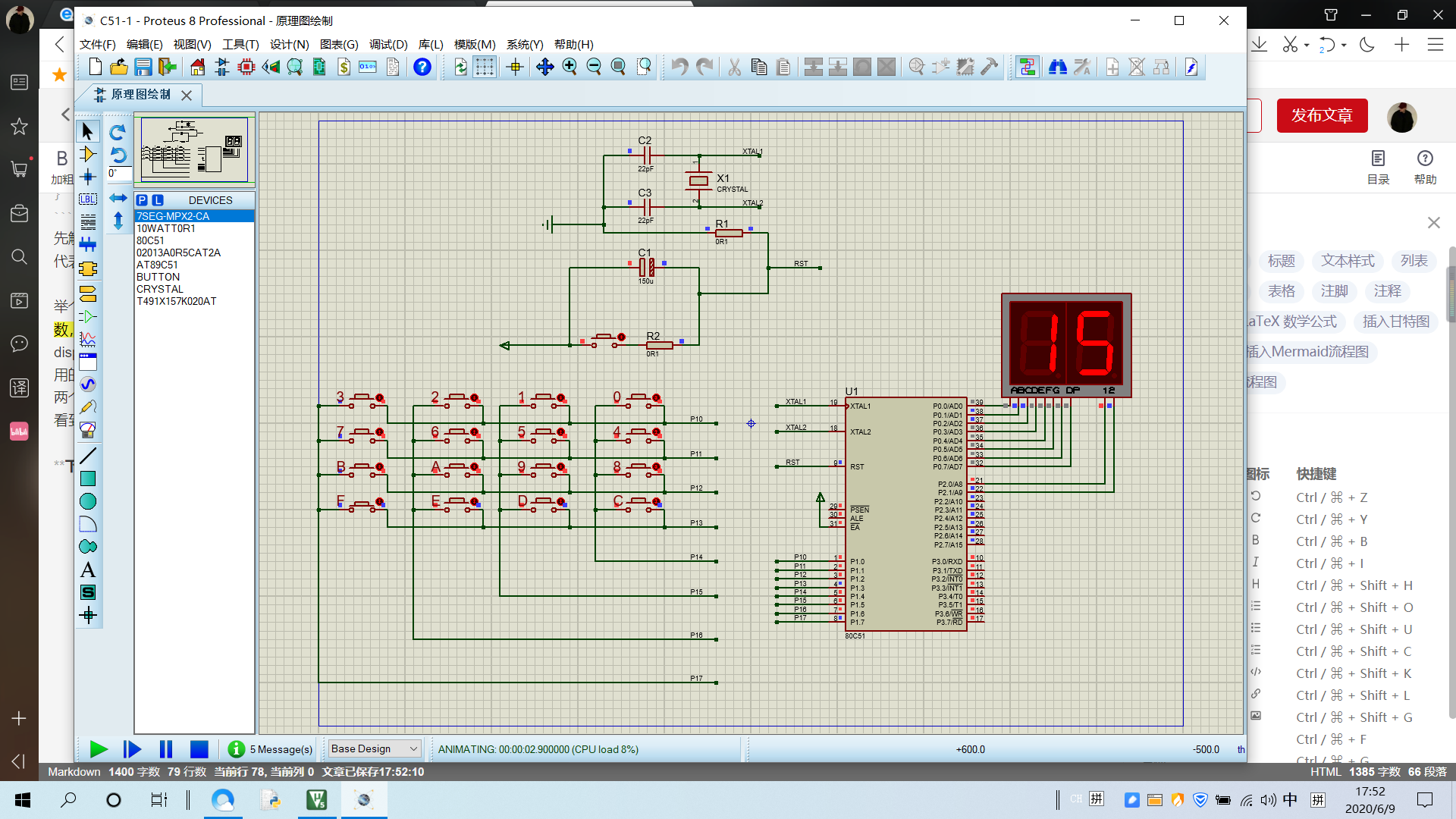# 我的第一个单片机程序！！！但是有些问题需要请教一下在座的各位大佬们

1 篇文章 0 订阅

#include <reg51.h>
#include <intrins.h>
#define  uchar  unsigned char
#define Time 50

sbit L1 = P1^0;
sbit L2 = P1^1;
sbit L3 = P1^2;
sbit L4 = P1^3;
sbit L5 = P1^4;
sbit L6 = P1^5;
sbit L7 = P1^6;
sbit L8 = P1^7;

uchar dis ={0xc0,0xf9,0xa4,0xb0,0x99,0x92,
0x82,0xf8,0x80,0x90};

unsigned  int  time;
void delay(time)     //delay_function
{
unsigned  int j,i;
for( j=0;j<80;j++)
for(i=0;i<time;i++);
}

void display(i,k)    //display_function
{
P2 = 0x01; P0 = dis[i];delay(Time);
P2 = 0x02; P0 = dis[k];delay(Time);
}

void judge(i,a,b,c,d)
{
if(L5 == 0) while(1) display(i,a);
if(L6 == 0) while(1) display(i,b);
if(L7 == 0) while(1) display(i,c);
if(L8 == 0) while(1) display(i,d);
}

main()
{
uchar temp,i;
while(1)
{
P1= 0xef;
for(i=0;i<4;i++)
{
if(L1 == 0)        //press first line
judge(0,0,1,2,3);
if(L2 == 0)        //press second line
judge(0,4,5,6,7);
if(L3 == 0)        //press third line
{
if(L5 == 0) while(1) display(0,8);
if(L6 == 0) while(1) display(0,9);
if(L7 == 0) while(1) display(1,0);
if(L8 == 0) while(1) display(1,1);
}
if(L4 == 0)        //press forth line
judge(1,2,3,4,5);
temp = P1;
temp = temp|0x0f;
temp = temp<<1;
temp = temp|0X0f;
P1 = temp;
}
}
}12-011795
12-02142711-282751
11-301214
12-022438
11-292003
11-301146
12-011529
11-205229
11-232030
11-291996
11-30191
12-03257
12-03115
11-291203
12-04292
12-032699
11-30221
11-232809
05-23996点击重新获取扫码支付余额充值